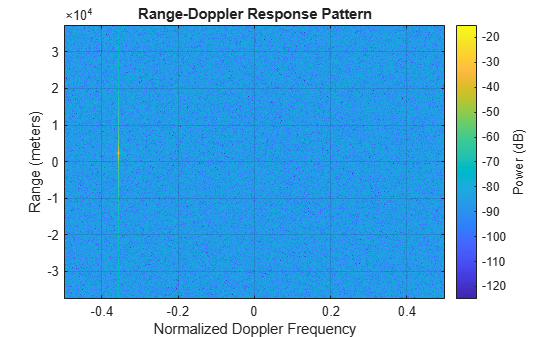# plotResponse

System object: phased.RangeDopplerResponse
Package: phased

Plot range-Doppler response

## Syntax

```plotResponse(H,x) plotResponse(H,x,xref) plotResponse(H,x,coeff) plotResponse(___,Name,Value) hPlot = plotResponse(___) ```

## Description

`plotResponse(H,x)` plots the range-Doppler response of the input signal, `x`, in decibels. This syntax is available when you set the `RangeMethod` property to `'FFT'` and the `DechirpInput` property to `false`.

`plotResponse(H,x,xref)` plots the range-Doppler response after performing a dechirp operation on `x` using the reference signal, `xref`. This syntax is available when you set the `RangeMethod` property to `'FFT'` and the `DechirpInput` property to `true`.

`plotResponse(H,x,coeff)` plots the range-Doppler response after performing a matched filter operation on `x` using the matched filter coefficients in `coeff`. This syntax is available when you set the `RangeMethod` property to `'Matched filter'`.

`plotResponse(___,Name,Value)` plots the angle-Doppler response with additional options specified by one or more `Name,Value` pair arguments.

`hPlot = plotResponse(___)` returns the handle of the image in the figure window, using any of the input arguments in the previous syntaxes.

## Input Arguments

 `H` Range-Doppler response object. `x` Input data. Specific requirements depend on the syntax: In the syntax `plotResponse(H,x)`, each column of the matrix `x` represents a dechirped signal from one frequency sweep. The function assumes all sweeps in `x` are consecutive.In the syntax `plotResponse(H,x,xref)`, each column of the matrix `x` represents a signal from one frequency sweep. The function assumes all sweeps in `x` are consecutive and have not been dechirped yet.In the syntax `plotResponse(H,x,coeff)`, each column of the matrix `x` represents a signal from one pulse. The function assumes all pulses in `x` are consecutive. In the case of an FMCW waveform with a triangle sweep, the sweeps alternate between positive and negative slopes. However, `phased.RangeDopplerResponse` is designed to process consecutive sweeps of the same slope. To apply `phased.RangeDopplerResponse` for a triangle-sweep system, use one of the following approaches: Specify a positive `SweepSlope` property value, with `x` corresponding to upsweeps only. In the plot, change the tick mark labels on the horizontal axis to reflect that the Doppler or speed values are half of what the plot shows by default.Specify a negative `SweepSlope` property value, with `x` corresponding to downsweeps only. In the plot, change the tick mark labels on the horizontal axis to reflect that the Doppler or speed values are half of what the plot shows by default. You can specify this argument as single or double precision. `xref` Reference signal, specified as a column vector having the same number of rows as `x`. You can specify this argument as single or double precision. `coeff` Matched filter coefficients, specified as a column vector. You can specify this argument as single or double precision.

### Name-Value Pair Arguments

Specify optional comma-separated pairs of `Name,Value` arguments. `Name` is the argument name and `Value` is the corresponding value. `Name` must appear inside quotes. You can specify several name and value pair arguments in any order as `Name1,Value1,...,NameN,ValueN`.

 `'NormalizeDoppler'` Set this value to `true` to normalize the Doppler frequency. Set this value to `false` to plot the range-Doppler response without normalizing the Doppler frequency. This parameter applies when you set the `DopplerOutput` property of `H` to `'Frequency'`. Default: `false` `'Unit'` The unit of the plot. Valid values are `'db'`, `'mag'`, and `'pow'`. Default: `'db'`

## Examples

expand all

Compute the range-Doppler response of an FMCW signal using an FFT.

Load data for an FMCW signal that has not been dechirped. The signal contains the return from a target about 2200 m away. The signal has a normalized Doppler frequency of approximately -0.36 relative to the radar.

`load RangeDopplerExampleData;`

Create a range-Doppler response object.

```hrdresp = phased.RangeDopplerResponse(... 'RangeMethod','FFT',... 'PropagationSpeed',RangeDopplerEx_Dechirp_PropSpeed,... 'SampleRate',RangeDopplerEx_Dechirp_Fs,... 'DechirpInput',true,... 'SweepSlope',RangeDopplerEx_Dechirp_SweepSlope);```

Plot the range-Doppler response.

```plotResponse(hrdresp,... RangeDopplerEx_Dechirp_X,RangeDopplerEx_Dechirp_Xref,... 'Unit','db','NormalizeDoppler',true)```